# Center of Mass

The terms "center of mass" and "center of gravity" are used synonymously in a uniform gravity field to represent the unique point in an object or system which can be used to describe the system's response to external forces and torques. The concept of the center of mass is that of an average of the masses factored by their distances from a reference point. In one plane, that is like the balancing of a seesaw about a pivot point with respect to the torques produced.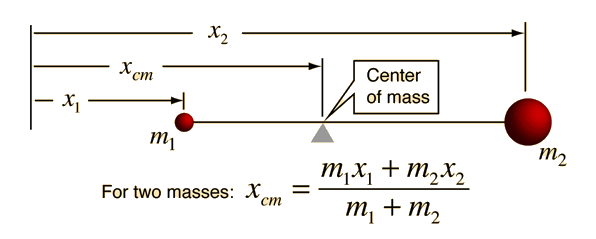If you are making measurements from the center of mass point for a two-mass system then the center of mass condition can be expressed aswhere r1 and r2 locate the masses. The center of mass lies on the line connecting the two masses.

 Center of mass for Collection of point masses Continuous mass distribution
 Determining center of mass of extended object

Index

Torque concepts

 HyperPhysics***** Mechanics ***** Rotation R Nave
Go Back

# Center of Mass for Particles

The center of mass is the point at which all the mass can be considered to be "concentrated" for the purpose of calculating the "first moment", i.e., mass times distance. For two masses this distance is calculated fromFor the more general collection of N particles this becomes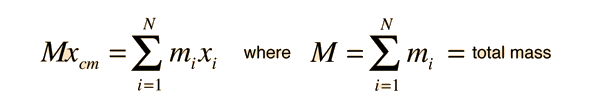and when extended to three dimensions: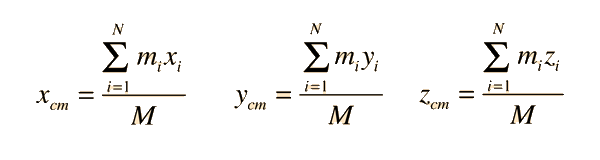This approach applies to discrete masses even if they are not point masses if the position xi is taken to be the position of the center of mass of the ith mass. It also points the way toward the calculation of the center of mass of an extended object.

Index

Torque concepts

 HyperPhysics***** Mechanics ***** Rotation R Nave
Go Back

# Center of Mass: Continuous

For a continuous distribution of mass, the expression for the center of mass of a collection of particles: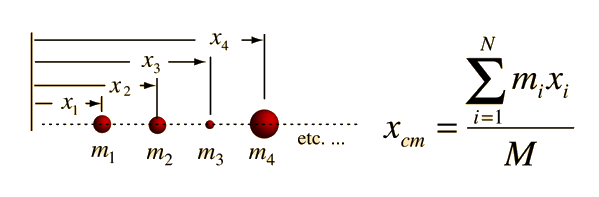becomes an infinite sum and is expressed in the form of an integralFor the case of a uniform rod this becomes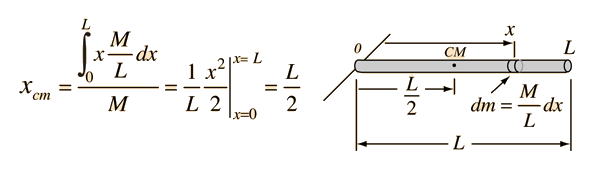This example of a uniform rod previews some common features about the process of finding the center of mass of a continuous body. Continuous mass distributions require calculus methods involving an integral over the mass of the object. Such integrals are typically transformed into spatial integrals by relating the mass to a distance, as with the linear density M/L of the rod. Exploiting symmetry can give much information: e.g., the center of mass will be on any rotational symmetry axis. The use of symmetry would tell you that the center of mass is at the geometric center of the rod without calculation.

Index

Torque concepts

 HyperPhysics***** Mechanics ***** Rotation R Nave
Go Back